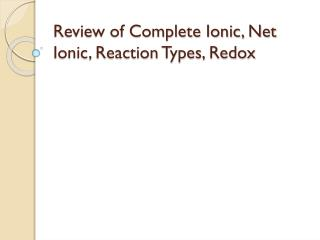DownloadDownload PresentationReview of Complete Ionic, Net Ionic, Reaction Types, Redox

# Review of Complete Ionic, Net Ionic, Reaction Types, Redox

Download Presentation## Review of Complete Ionic, Net Ionic, Reaction Types, Redox

- - - - - - - - - - - - - - - - - - - - - - - - - - - E N D - - - - - - - - - - - - - - - - - - - - - - - - - - -
##### Presentation Transcript

1. Review of Complete Ionic, Net Ionic, Reaction Types, Redox

2. What Do You Call Santa's Helpers? Subordinate Clauses.

3. Which of the following is a double replacement reaction? • 2Al + 3H2SO4 Al2(SO4)3 + 3H2 • Na2SO4 + 2AgNO3Ag2SO4 + 2NaNO3 • 3C + Fe2O3 2Fe + 3CO • All are double replacement reactions

4. A double replacement reaction will occur if • All the products are soluble • One of the products formed is NaCl • One of the products is insoluble • One of the products is NaOH • None of the answers is right

5. Which of the following is insoluble? • Na2SO4 • KSO4 • FeSO4 • PbSO4 • All are soluble

6. Which of the following is insoluble? • SrBr2 • Sr(C2H3O2)2 • SrCO3 • SrCl2 • All are soluble

7. Which of the following is insoluble? • PbCl2 • SrCl2 • CaCl2 • BaCl2 • All are soluble

8. Which of the following would be insoluble? • NaCl • KBr • Ca(NO3)2 • BaSO4 • All are soluble

9. Which of the following is insoluble? • FeS • Ca(ClO3)2 • FeSO4 • NaNO3 • All are soluble

10. KCl + Hg(NO3)2 • KNO3 + HgCl2 • KNO3 + HgCl • KNO3 + H2O + Hg • KHg + NO3(OH)2 • Not listed

11. Based on the products will the reaction occur between KCl + Hg(NO3)2 ? • Yes • No

12. KCl + Hg(NO3)2 What would be the precipitate if any? • KNO3 • HgCl • HgCl2 • KHg • Not listed

13. KCl + Hg(NO3)2 What would be one of the ions in solution after the reaction? • Cl- • Hg2+ • NO3+ • K2+ • Not listed

14. Write the balanced chemical equation, complete ionic and net ionic equation for the following reaction?Potassium chromate reacting with barium nitrate 

15. The test won’t be this bad!!!

16. Complete Ionic Equations Molecular Equation: K2CrO4 + Ba(NO3)2 BaCrO4 + 2 KNO3 Soluble Soluble Insoluble Soluble Complete Ionic Equation: 2 K+ + CrO4-2+ Ba+2 + 2 NO3- BaCrO4 (s) + 2 K+ + 2 NO3-

17. Net Ionic Equations • These are the same as complete ionic equations, but you should cancel out ions that appear on BOTH sides of the equation Complete Ionic Equation: 2 K+ + CrO4-2 + Ba+2 + 2 NO3-  BaCrO4 (s) + 2 K+ + 2 NO3- Net Ionic Equation: CrO4-2 + Ba+2  BaCrO4 (s) Spectator Ion

18. Which of the following reaction(s) are redox reaction(s)? • 1, 2, 3 only • 1, 2, 3, 4 • 1 and 4 only • 1, 3, 4 only 1. 2Al + 3H2SO4Al2(SO4)3 + 3H22. Na2SO4 + 2AgNO3Ag2SO4 + NaNO33. 3C + Fe2O3 2Fe + 3CO 4. H2 + F2 2HF

19. In the following reaction, H is…H2 + CuO Cu + H2O • Oxidized • Reduced • Neither oxidized nor reduced • Both oxidized and reduced

20. In the following reaction, H is…CH4 + 2O2  CO2 + 2H2O • Oxidized • Reduced • Neither oxidized nor reduced • Both oxidized and reduced

21. In the following reaction, Cl is…H2 + Cl2 2HCl • Oxidized • Reduced • Neither oxidized nor reduced • Both oxidized and reduced

22. In the following reaction, Na is…2NaOH + H2SO4  2H2O + Na2SO4 • Oxidized • Reduced • Neither oxidized nor reduced • Both oxidized and reduced

23. The equation 2C2H6 + 7O2 4CO2 + 6H2O is an example of an combustion reaction. The hydrogen is a +1 on both sides of the equation. Is this a redox reaction? • No • Yes, O is oxidized and H is reduced. • Yes, C is reduced and O is oxidized. • Yes, C is oxidized and H is reduced. • Yes, C is oxidized and O is reduced.

24. CaCO3 → • Double Replacement • Synthesis • Decomposition • Single Replacement • Combustion

25. Al + O2 • AlO2 • Al2O3 • AlO3 • Al3O2 • CO2 + H2O • Not listed

26. Fe + NaBr • FeBr + Na • FeNa + Br2 • NaBr2 + Fe • No Reaction

27. KBr • K + Br • KBr • K + Br2 • K2 + Br2 • Not Listed